# Beginner's Guide to Statistics and Probability Distribution

We have all realised that a working knowledge of statistics is essential for modelling different strategies when it comes to algorithmic trading. In fact, data science, one of the most sought after skills in this decade, employs statistics to model data and arrive at meaningful conclusions. With that aim in mind we will go through some basic terminologies as well as the types of probability distributions which are employed in the domain of algorithmic trading.

We will go through the following topics:

## Historical Data Analysis

In this section, we will try to answer the fundamental question, “How do you analyse a stock’s historical data and use it for strategy building?” Of course, for the analysis, we first need a data set!

### Dataset

In order to keep it universal, we have taken the daily stock price data of Apple, Inc. from Dec 26, 2018, to Dec 26, 2019. You can download historical data from Yahoo Finance. If you are interested in downloading the data using python, you can visit the following link.

For the time being, we will use the following python code to download it from yahoo finance;

import yfinance as yf
aapl = yf.download('AAPL','2018-12-26', '2019-12-26')

This is a time series data set with daily closing prices and volumes for Apple. We’ll base our analysis on the closing prices for this stock. We’ll just touch upon the basic statistical properties for the daily stock prices in this post, which would be followed by a brief on correlation.

Mean? Mode? Median? What’s the difference!!!

We will just take 5 numbers as an example: 12, 13, 6, 7, 19, 21, and understand the three terms.

### Mean

To put it simply, mean is the one we are most used to, i.e. the average. Thus, in the above example, the mean = (12 + 13 + 6 + 7 + 19 + 21)/6 = 13.

In the AAPL dataset, the mean of closing prices is 204.84. The rolling mean is a widely used measure in technical trading strategies. The traders place great importance to cross over of 50 days and 200 days rolling mean. And initiate trade based on it.

For the AAPL dataset, we will use the following python code:

mean = np.mean(aapl['Adj Close'])
mean

The output is:
204.84638595581055

### Mode

In a given dataset, the mode will be the number which is occurring the most. In the above example, since there is no value which is repeated, there is no mode. You can argue that every element is a mode. But that doesn't help in summarizing the dataset.

In the AAPL dataset, the mode of closing prices does not exist as there is no repeating value.

When we try to run the following code to find the mode in python, it throws the following error

import statistics
mode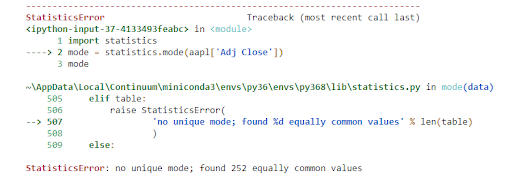Also, if your dataset is as below, which value of mode you will go with?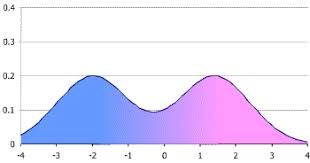It is difficult to answer that question, and some other measure should be used. Also, the mode doesn’t really make much sense for closing prices or other continuous data. A mode is especially useful when you want to plot histograms and visualize the frequency distribution.

### Median

Sometimes, the data set values can have a few values which are at the extreme ends, and this might cause the mean of the data set to portray an incorrect picture. Thus, we use the median, which gives the middle value of the sorted data set.

To find the median, you have to arrange the numbers in ascending order and then find the middle value. If the dataset contains an even number of values, you take the mean of the middle two values. In our example, the median is (12 + 13)/2 = 12.5

In our data set, the median of the closing price is 201.05

The python code for finding the median is the following:

median = np.median(aapl['Adj Close'])

Great! We now move on to a term which is very important when we start learning about statistics, i.e. Probability distribution.

## Probability distribution

We have all gone through the example of finding the probabilities of a dice roll. Now, we know that there are only six outcomes on a dice roll, i.e. {1, 2, 3, 4, 5, 6}. The probability of rolling a 1 is 1/6. This kind of probability is called discrete, where there are a fixed number of outcomes.

Now, as the name suggests, the probability distribution is simply a list of all outcomes of a given event. Thus, the probability of the dice roll event is the following:

 Dice roll number Probability Distribution 1 1/6 2 1/6 3 1/6 4 1/6 5 1/6 6 1/6

Listing all the values here works because we have a limited set of outcomes, but if the outcomes are large, we use functions.

If the probability is discrete, we call the function a probability mass function. In the case of dice roll, it will be P(x) = 1/6 where x = {1,2,3,4,5,6}.

For discrete probabilities, there are certain cases which are so extensively studied, that their probability distribution has become standardised. Let’s take, for example, Bernoulli's distribution, which takes into account the probability of getting heads or tails when we toss a coin. We write its probability function as px (1 – p)(1 – x). Here x is the outcome, which could be written as heads = 0 and tails = 1.

Now, there are cases where the outcomes are not clearly defined. For example, the heights of all high school students in one grade. While the actual reason is different, we can say that it will be too cumbersome to list down all the height data and the probability. It is in this situation that the functions are essential.

Earlier, we said that for discrete values, the probability function is the probability mass function. In comparison, for continuous values, the probability function is known as a probability density function.

Let us take a step back and understand some terms related to the probability distribution.

### Range

Range simply gives the difference between the min and max values of the data set.

In the data set taken, the minimum value of the closing price is 140.08 while the maximum value is 284.26. Thus, the range = 284.26 - 140.08 = 144.18. Now, we will move towards standard deviation.

In python, we can find the values by a simple line of code:

aapl['Adj Close'].describe()

The output is as follows: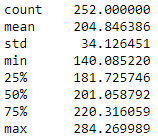### Standard Deviation

In simple words, the standard deviation tells us how far the value deviates from the mean. Let us use the full dataset and try to understand how the standard deviation helps us in the arena of trading.

We are taking into account the closing price for our calculations. As specified previously, the mean of our dataset is 204.84. The python code for Plotting the graph with the closing price and the mean should give us the following figure.

import matplotlib.pyplot as plt
plt.axhline(y=mean, color='r', linestyle='-')
plt.legend()
plt.grid()
plt.show()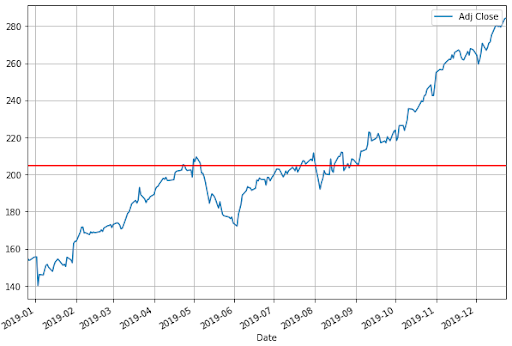The standard deviation is calculated as:

1. Calculate the simple average of the numbers (mean)
2. Subtract the mean from each number
3. Square the result
4. Calculate the average of the results
5. Take square root of the answer in step 4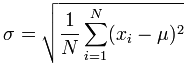For the data set given, the code is as follows:

std = np.std(aapl['Adj Close'])

The standard deviation of the closing price would be 34.05.

Now we will plot the above graph with one standard deviation on both sides of the mean. We will write it as (+S.D.) = 204.84 + 34.05 = 238.89, and (-S.D.) = 204.84 - 34.05 = 170.79.

The code is as follows:

aapl['Adj Close'].plot(figsize=(10,7))
plt.axhline(y=mean, color='r', linestyle='-')
plt.axhline(y=mean+std, color='r', linestyle='-')
plt.axhline(y=mean-std, color='r', linestyle='-')
plt.legend()
plt.grid()
plt.show()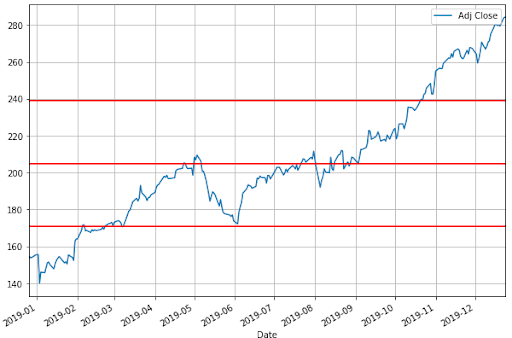In the graph, the mean is shown as the middle red line while the +S.D. and -S.D. are the other red lines.

So tell us, what can you observe by looking at the above graph?

Well, a quick look tells us that most of the closing price values are in between the two standard deviations. Thus, this gives us a rough idea about the majority of the price action trading

But you might still be wondering, what is the use of knowing a certain range of price values? Well, for one thing, standard deviation plays an important role in Bollinger Bands, which is a quite popular indicator. You can use the upper standard deviation as a sign of a breakout. And initiate a buy trade when the price moves above the upper band.

The volatility of the stock can be calculated using the standard deviation. The stock volatility is an important feature used in many machine learning algorithms. It is also used in Normal probability distribution, which we will cover in a while.

Wait! Normal distribution?

Normal distribution is a very simple and yet, quite profound piece in the world of statistics, actually in general life too. The basic premise is that given a range of observations, it is found that most of the values centre around the mean and within one standard deviation away from the mean. Actually, it is said that 68% of the values are within this range. If we move ahead, then we see 95% of the values within two standard deviations from the mean.

Wait, we are going ahead of ourselves now. Let us first take the help of something called the histogram to understand this.

### Histogram

Let’s take an example of the heights of students in a batch. Now there might be students who have heights of 60.1 inch, 60.2 inches and so on till 60.9. Sometimes we are not looking for that level of detail and would like just to find out how many students have a height of 60 - 61 inches. Wouldn’t that make our job easier and simpler? That is exactly what a histogram does. It gives us the frequency distribution of the observed values.

When it comes to trading, we usually use the daily percentage change instead of the closing prices.

For our dataset, we will use the following code:

aapl['daily_percent_change'] = aapl['Adj Close'].pct_change()
aapl.daily_percent_change.hist(figsize=(10,7))
plt.ylabel('Frequency')
plt.xlabel('Daily Percentage Change')
plt.show()

The output is as follows: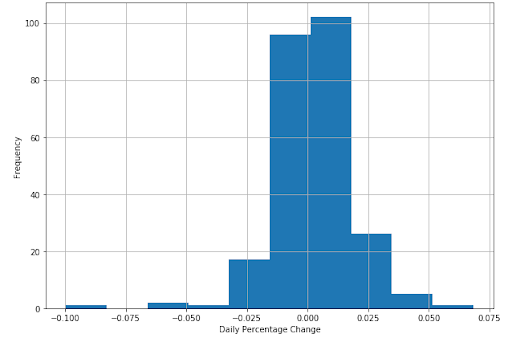Recall how we said that the majority of the values are situated close to the mean. You can see it clearly in the histogram plotted above.

In fact, if we draw a line curve around the values, it would look like a bell.

We call this a bell curve, which is another name for the normal probability distribution, or normal distribution for short. You can see the majority of the values lying between the standard deviations, i.e. (+S.D.) = 239.6, and (-S.D.) = 172.64.

You might want to keep in mind that in a normal distribution, 68% of the values lie between one standard deviation and 95% of the values lie between two standard deviations. Moving further, we will say that 99.7% of the values lie between 3 standard deviations of the mean.

### Normal distribution

When the distribution of your data meets certain requirements, such as symmetry around the mean and bell-shaped curve, we say your data is normally distributed.

Statistically speaking, if X is Normally distributed with mean µ and standard deviation σ, we write X∼N(µ, σ^2), where µ and σ are the parameters of the distribution.

#### Why is it useful to know the distribution function of your dataset?

If you know that your data sample is, say, normally distributed, you can make ‘predictions’ about your population with certain ‘confidence’.

For example, say, your data sample X represents marks obtained out of 100 in an entrance test for a sample of students. The data is normally distributed, such as X∼N(50, 102). When plotted, this data would look as follows: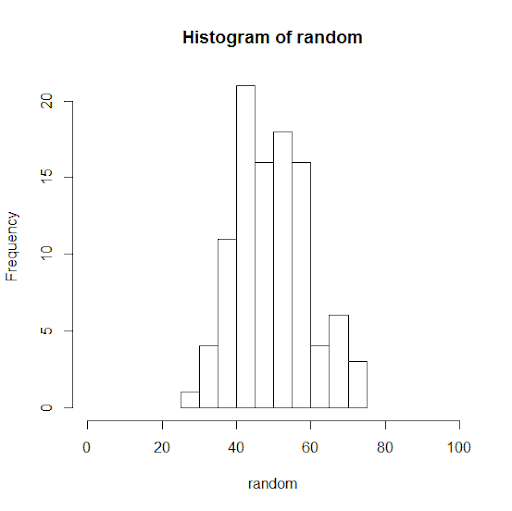If you increase the number of observations in your sample data set from 100 to 1000, this is what happens: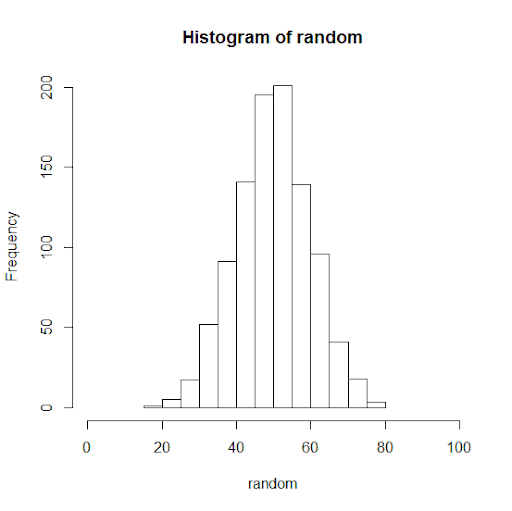It looks more bell-shaped!

Now that we know, X has normally distributed data with mean at 50 and standard deviation of 10, we can predict the marks of the entire student population or future students (from the same population) with a certain confidence. With almost 99.7% confidence, we can say that students would not get less than 20 or greater than 80 marks. With 95% confidence, we can say that students would get marks between 30 and 70 points.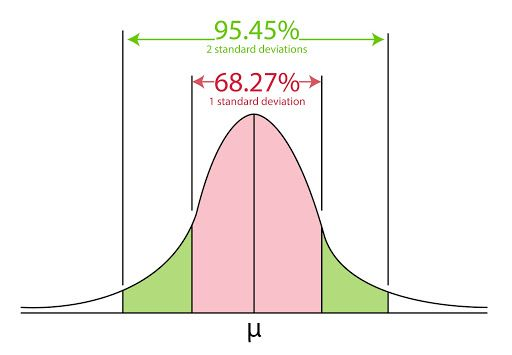Statistically speaking, distribution functions give us the probability of expecting the value of a given observation between two points. Hence, using distribution functions, also called probability density functions, we can ‘predict’ with certain ‘confidence’.

### Are closing prices normally distributed?

Before we try to answer the question, let us take another dataset and see how its histogram looks like.

We have plotted the histogram of Tesla, Inc. for the same period and see the following: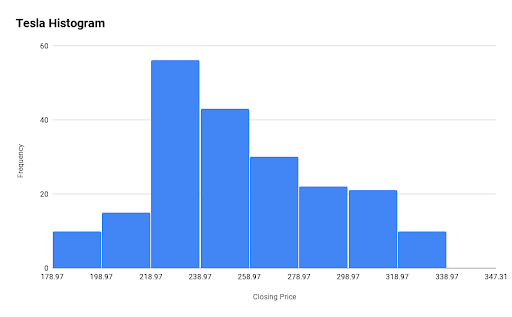Here, the mean(Closing price) is 270.9 and the +S.D. and -S.D. is 319.14 and 222.66 respectively. So what conclusion can you draw from the above histogram?

To sum it up, probability distribution functions are used in every step of technical analysis, and it is the core of the quantitative analysis. These analyses constitute the core part of any strategy building process.

So far, we have gone through some basic concepts in the world of statistics. Now, we shall try to go a bit further in this fascinating world and see its application in trading. We will first start with correlation.

## Correlation

Am I related to you or not?

Well, in a way, correlation tells us about the relationship between two sets of values. Until now, we have taken the data set of Apple from Dec 26, 2018 to Dec 26, 2019. Now, we should point that Apple is part of the S&P 500 index. Thus, any change in Apple stocks could in some way reflect on the S&P index.

Let us take the dataset of the S&P500 for the same time period and find the correlation.

The code is as follows:

import yfinance as yf
plt.figure(figsize=(10,7))
plt.scatter(aapl.daily_percent_change,spx.daily_percent_change)
plt.xlabel('AAPL Daily Returns')
plt.ylabel('SPY Daily Returns')
plt.grid()
plt.show()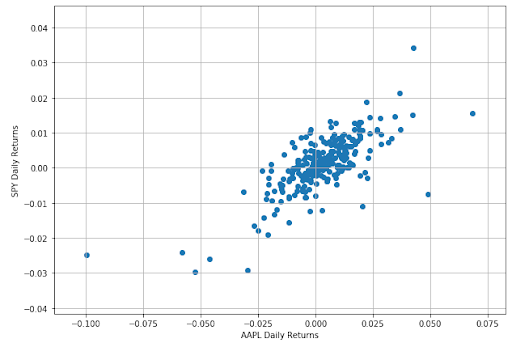from scipy.stats import spearmanr
correlation, _ = spearmanr(aapl.daily_percent_change.dropna(),spx.daily_percent_change.dropna())
print('Spearmans correlation is %.2f' % correlation)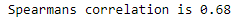### Understanding correlation

Correlation is a unit free number lying between -1 and 1 which gives us the measurement of the relationship between variables. A highly positive correlation value lying between 0.7 and 1.0 means that the change in one variable is positively related to the change in the other variable. That means, if one variable increases, there is a high probability that other one will increase as well. The behavior will be consistent in other cases of decrease or no change in value as well.

On the other hand, a highly negative correlation value lying between -0.7 to -1.0 tells us that the change in one variable is negatively related to the change in the other variable. That means, if one variable increase, there is a high probability that the other one will decrease.

The low correlation value around -0.2 and 0.2 tells us that there is no strong relationship between the two variables.

A point to note is that correlation doesn’t tell us anything about causality. We have all heard of the statement, “Correlation doesn’t imply causation”. For instance, it is possible that instances of lung cancers are correlated with the number of cigarettes smoked in a lifetime among a population, that does not establish causality of smoking to lung cancer. One would be required to do a controlled group study keeping constant all other influential factors to establish such a causality relation. Machine learning based trading models are very good at extracting such causality between different indicators.

Correlation is the measure of the linear relationship. For instance, the correlation between x and x2 might be as close as 0. Even though there is a strong relationship between the two variables, it would not be captured in the correlation value.

Great! We have gone through a lot of concepts related to Statistics. You can move further to regression; in fact, the blog on Linear regression is a perfect next step in your quest to master the art of algorithmic trading.

## Conclusion

We have gone through basic concepts of mean, median and mode and then understood the probability distribution of discrete as well as continuous variables. We looked into normal distribution in detail and touched upon the topic of correlation to figure out if two datasets are related or not.

If you want to learn various aspects of Algorithmic trading and automated trading systems, then check out the Executive Programme in Algorithmic Trading (EPAT®). The course covers training modules like Statistics & Econometrics, Financial Computing & Technology, and Algorithmic & Quantitative Trading. EPAT® equips you with the required skill sets to build a promising career in algorithmic trading. Enroll now!

Disclaimer: All data and information provided in this article are for informational purposes only. QuantInsti® makes no representations as to accuracy, completeness, currentness, suitability, or validity of any information in this article and will not be liable for any errors, omissions, or delays in this information or any losses, injuries, or damages arising from its display or use. All information is provided on an as-is basis.